Clustering Georg Gerber Lecture 6 2602 Lecture Overview

• Slides: 50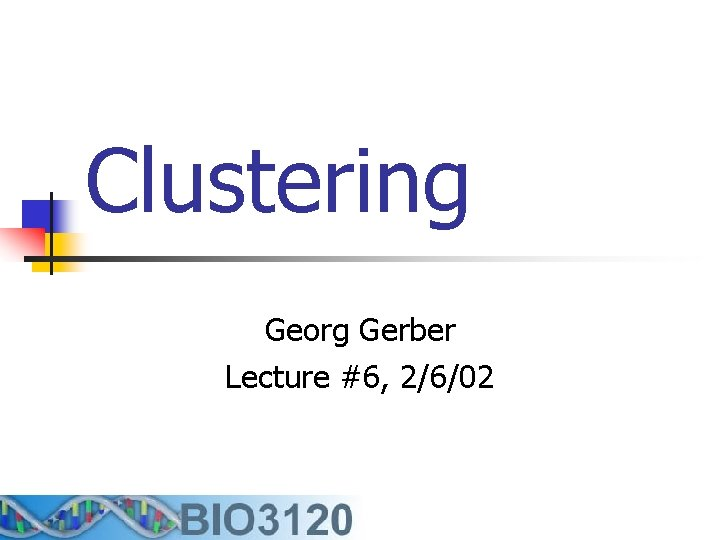Clustering Georg Gerber Lecture #6, 2/6/02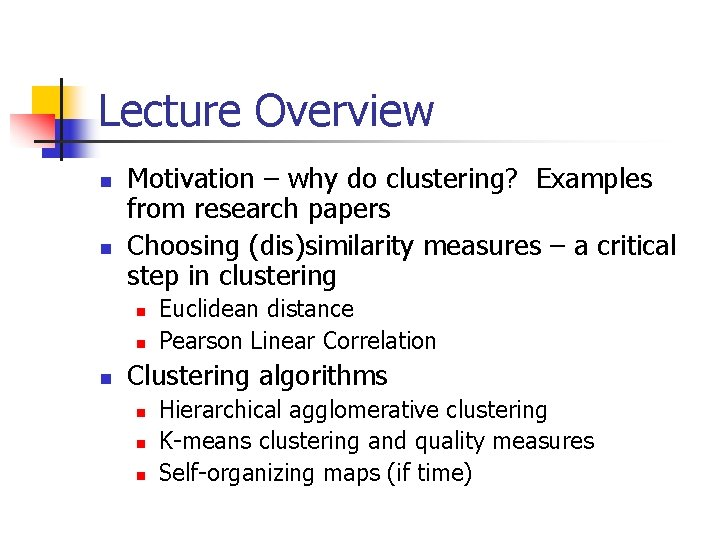Lecture Overview n n Motivation – why do clustering? Examples from research papers Choosing (dis)similarity measures – a critical step in clustering n n n Euclidean distance Pearson Linear Correlation Clustering algorithms n n n Hierarchical agglomerative clustering K-means clustering and quality measures Self-organizing maps (if time)What is clustering? n n n A way of grouping together data samples that are similar in some way - according to some criteria that you pick A form of unsupervised learning – you generally don’t have examples demonstrating how the data should be grouped together So, it’s a method of data exploration – a way of looking for patterns or structure in the data that are of interest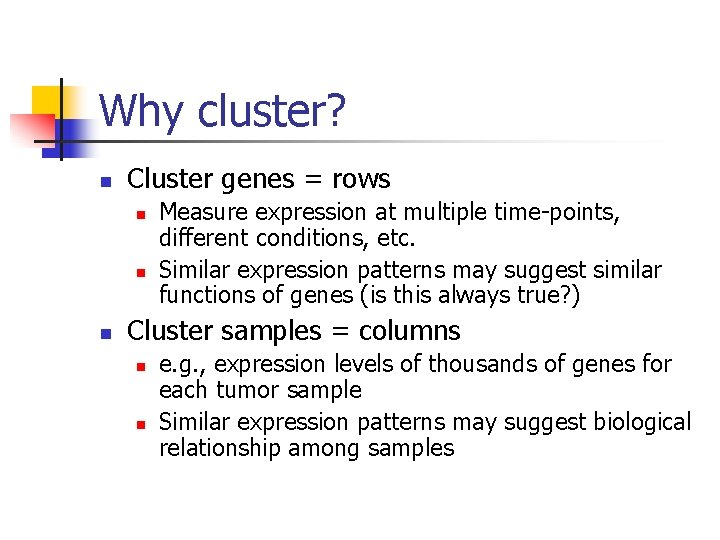Why cluster? n Cluster genes = rows n n n Measure expression at multiple time-points, different conditions, etc. Similar expression patterns may suggest similar functions of genes (is this always true? ) Cluster samples = columns n n e. g. , expression levels of thousands of genes for each tumor sample Similar expression patterns may suggest biological relationship among samples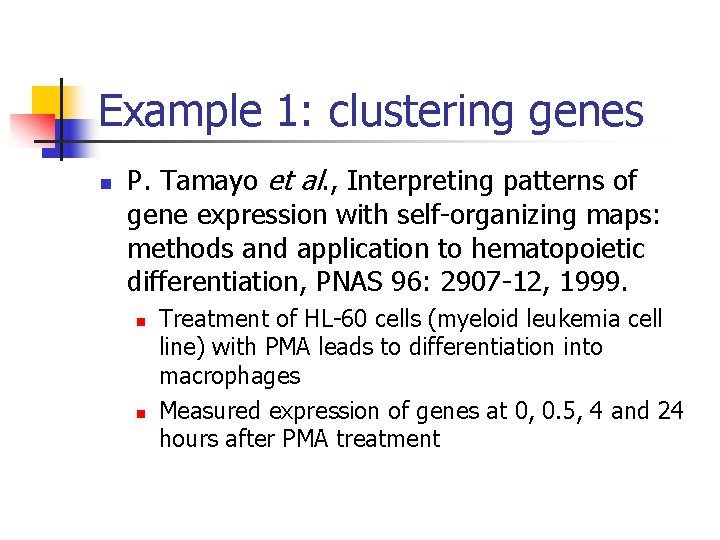Example 1: clustering genes n P. Tamayo et al. , Interpreting patterns of gene expression with self-organizing maps: methods and application to hematopoietic differentiation, PNAS 96: 2907 -12, 1999. n n Treatment of HL-60 cells (myeloid leukemia cell line) with PMA leads to differentiation into macrophages Measured expression of genes at 0, 0. 5, 4 and 24 hours after PMA treatment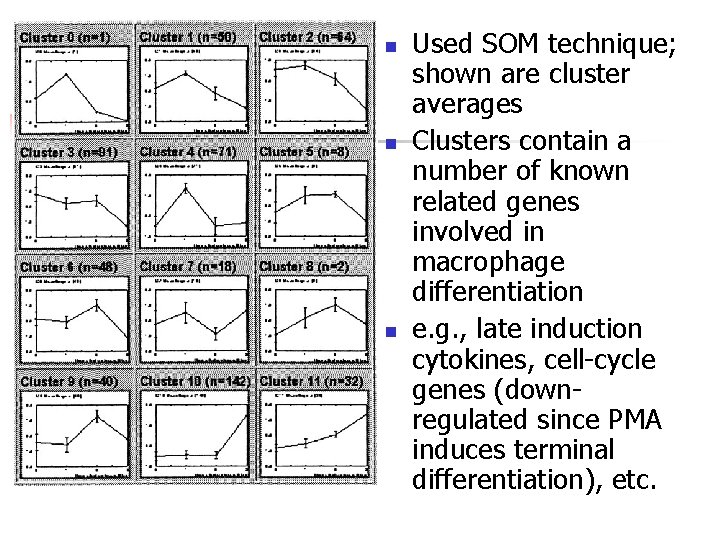n n n Used SOM technique; shown are cluster averages Clusters contain a number of known related genes involved in macrophage differentiation e. g. , late induction cytokines, cell-cycle genes (downregulated since PMA induces terminal differentiation), etc.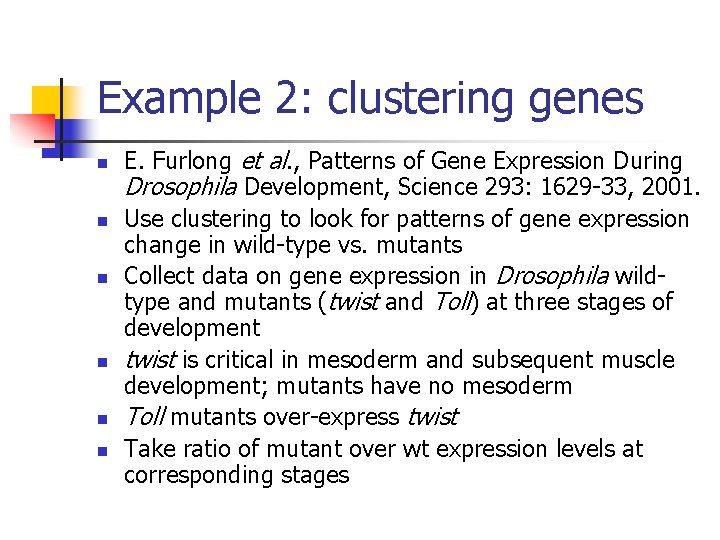Example 2: clustering genes n n n E. Furlong et al. , Patterns of Gene Expression During Drosophila Development, Science 293: 1629 -33, 2001. Use clustering to look for patterns of gene expression change in wild-type vs. mutants Collect data on gene expression in Drosophila wildtype and mutants (twist and Toll) at three stages of development twist is critical in mesoderm and subsequent muscle development; mutants have no mesoderm Toll mutants over-express twist Take ratio of mutant over wt expression levels at corresponding stagesFind general trends in the data – e. g. , a group of genes with high expression in twist mutants and not elevated in Toll mutants contains many known neuroectodermal genes (presumably overexpression of twist suppresses ectoderm)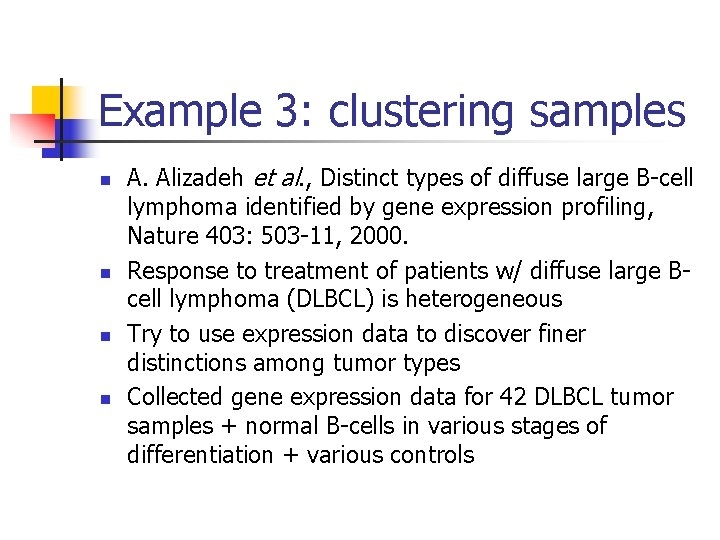Example 3: clustering samples n n A. Alizadeh et al. , Distinct types of diffuse large B-cell lymphoma identified by gene expression profiling, Nature 403: 503 -11, 2000. Response to treatment of patients w/ diffuse large Bcell lymphoma (DLBCL) is heterogeneous Try to use expression data to discover finer distinctions among tumor types Collected gene expression data for 42 DLBCL tumor samples + normal B-cells in various stages of differentiation + various controls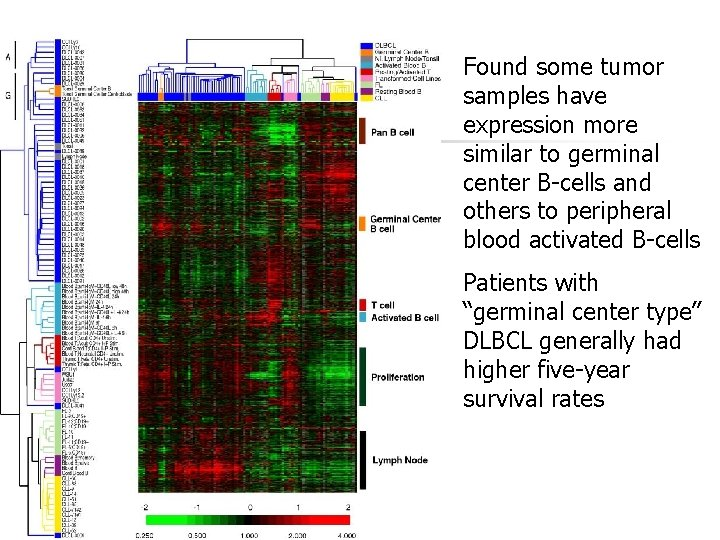Found some tumor samples have expression more similar to germinal center B-cells and others to peripheral blood activated B-cells Patients with “germinal center type” DLBCL generally had higher five-year survival rates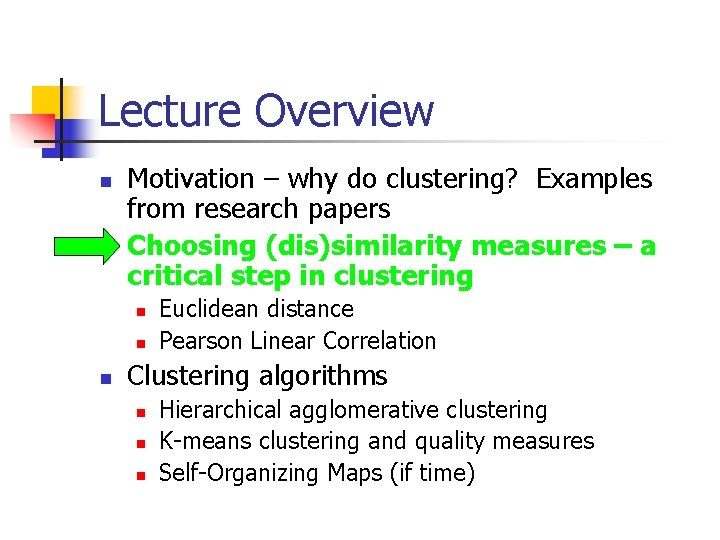Lecture Overview n n Motivation – why do clustering? Examples from research papers Choosing (dis)similarity measures – a critical step in clustering n n n Euclidean distance Pearson Linear Correlation Clustering algorithms n n n Hierarchical agglomerative clustering K-means clustering and quality measures Self-Organizing Maps (if time)How do we define “similarity”? n n n Recall that the goal is to group together “similar” data – but what does this mean? No single answer – it depends on what we want to find or emphasize in the data; this is one reason why clustering is an “art” The similarity measure is often more important than the clustering algorithm used – don’t overlook this choice!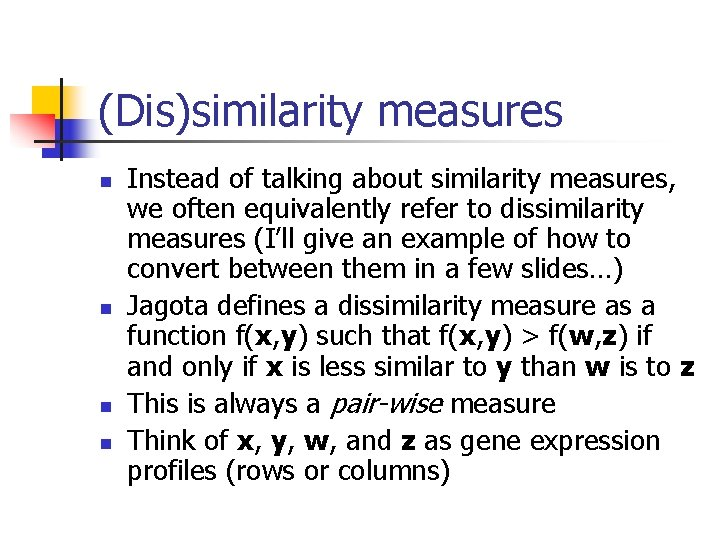(Dis)similarity measures n n Instead of talking about similarity measures, we often equivalently refer to dissimilarity measures (I’ll give an example of how to convert between them in a few slides…) Jagota defines a dissimilarity measure as a function f(x, y) such that f(x, y) > f(w, z) if and only if x is less similar to y than w is to z This is always a pair-wise measure Think of x, y, w, and z as gene expression profiles (rows or columns)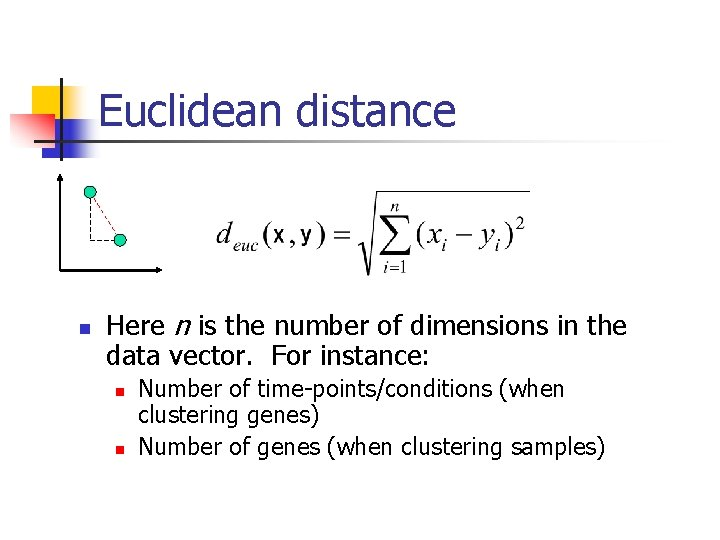Euclidean distance n Here n is the number of dimensions in the data vector. For instance: n n Number of time-points/conditions (when clustering genes) Number of genes (when clustering samples)deuc=0. 5846 deuc=1. 1345 deuc=2. 6115 These examples of Euclidean distance match our intuition of dissimilarity pretty well…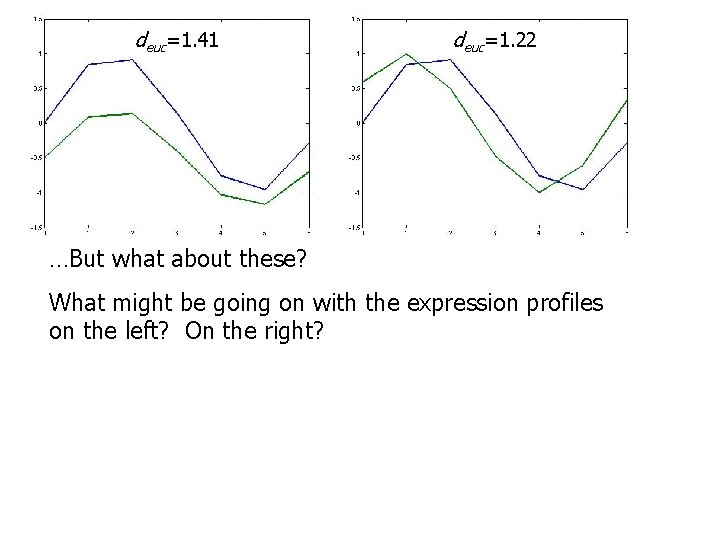deuc=1. 41 deuc=1. 22 …But what about these? What might be going on with the expression profiles on the left? On the right?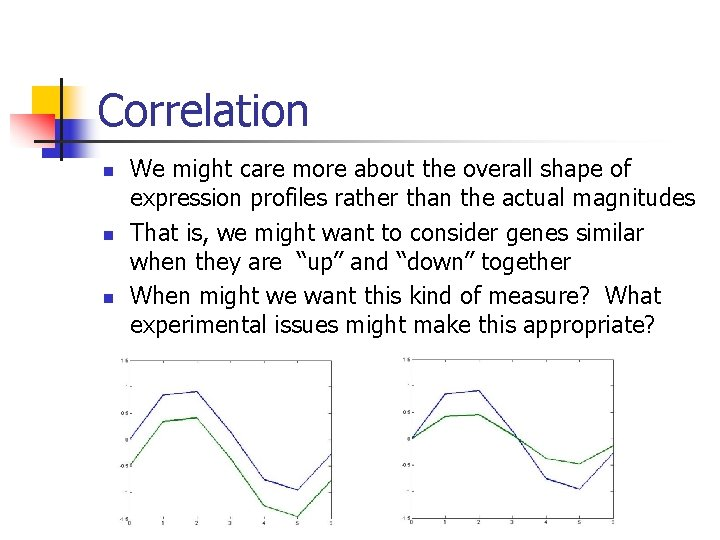Correlation n We might care more about the overall shape of expression profiles rather than the actual magnitudes That is, we might want to consider genes similar when they are “up” and “down” together When might we want this kind of measure? What experimental issues might make this appropriate?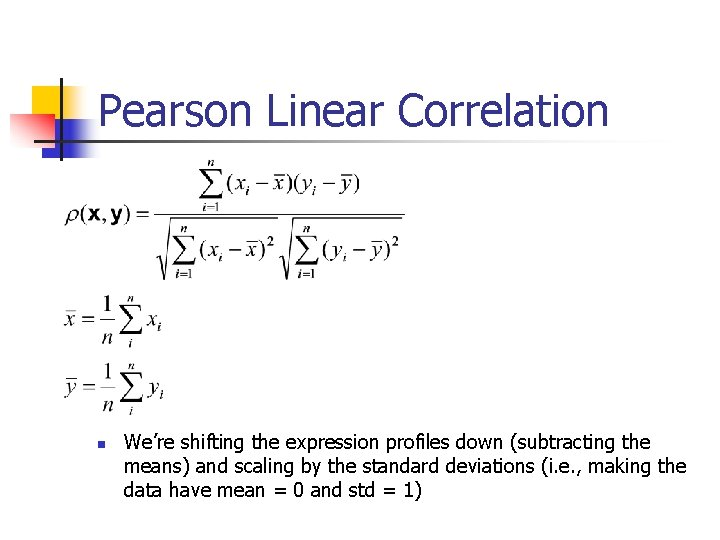Pearson Linear Correlation n We’re shifting the expression profiles down (subtracting the means) and scaling by the standard deviations (i. e. , making the data have mean = 0 and std = 1)Pearson Linear Correlation n Pearson linear correlation (PLC) is a measure that is invariant to scaling and shifting (vertically) of the expression values Always between – 1 and +1 (perfectly anti-correlated and perfectly correlated) This is a similarity measure, but we can easily make it into a dissimilarity measure:PLC (cont. ) n n PLC only measures the degree of a linear relationship between two expression profiles! If you want to measure other relationships, there are many other possible measures (see Jagota book and project #3 for more examples) = 0. 0249, so dp = 0. 4876 The green curve is the square of the blue curve – this relationship is not captured with PLCMore correlation examples What do you think the correlation is here? Is this what we want? How about here? Is this what we want?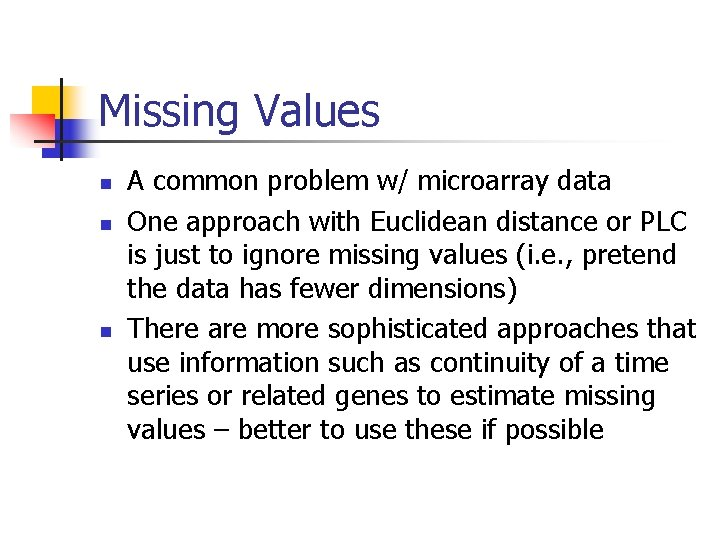Missing Values n n n A common problem w/ microarray data One approach with Euclidean distance or PLC is just to ignore missing values (i. e. , pretend the data has fewer dimensions) There are more sophisticated approaches that use information such as continuity of a time series or related genes to estimate missing values – better to use these if possibleMissing Values (cont. ) The green profile is missing the point in the middle If we just ignore the missing point, the green and blue profiles will be perfectly correlated (also smaller Euclidean distance than between the red and blue profiles)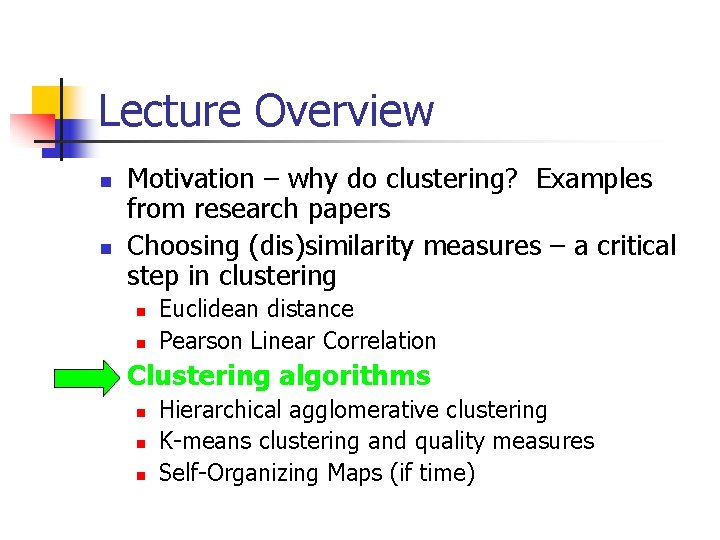Lecture Overview n n Motivation – why do clustering? Examples from research papers Choosing (dis)similarity measures – a critical step in clustering n n n Euclidean distance Pearson Linear Correlation Clustering algorithms n n n Hierarchical agglomerative clustering K-means clustering and quality measures Self-Organizing Maps (if time)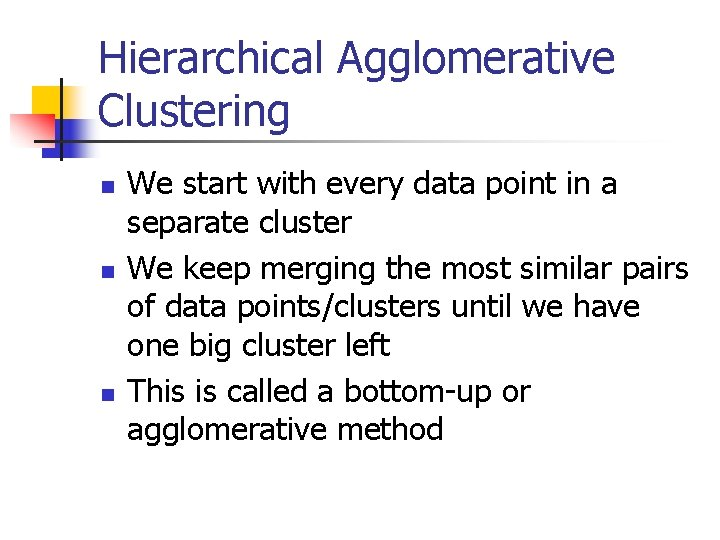Hierarchical Agglomerative Clustering n n n We start with every data point in a separate cluster We keep merging the most similar pairs of data points/clusters until we have one big cluster left This is called a bottom-up or agglomerative methodHierarchical Clustering (cont. ) n This produces a binary tree or dendrogram n n The final cluster is the root and each data item is a leaf The height of the bars indicate how close the items areHierarchical Clustering Demo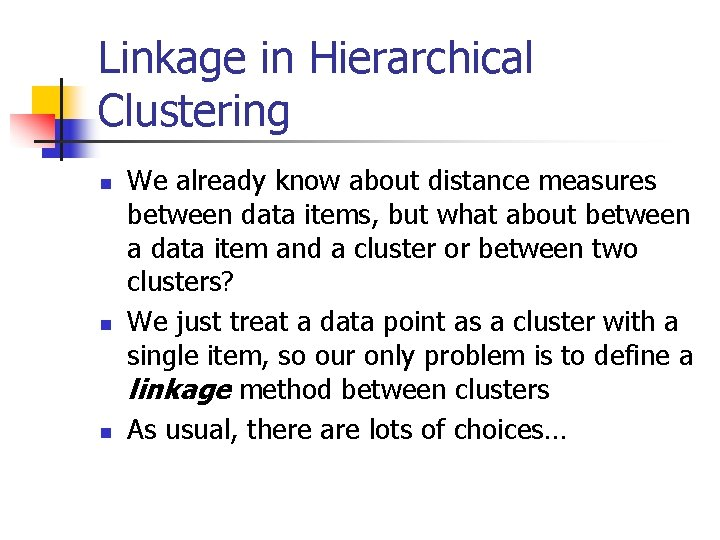Linkage in Hierarchical Clustering n n n We already know about distance measures between data items, but what about between a data item and a cluster or between two clusters? We just treat a data point as a cluster with a single item, so our only problem is to define a linkage method between clusters As usual, there are lots of choices…Average Linkage n Eisen’s cluster program defines average linkage as follows: n n n Each cluster ci is associated with a mean vector i which is the mean of all the data items in the cluster The distance between two clusters ci and cj is then just d( i , j ) This is somewhat non-standard – this method is usually referred to as centroid linkage and average linkage is defined as the average of all pairwise distances between points in the two clustersSingle Linkage n n The minimum of all pairwise distances between points in the two clusters Tends to produce long, “loose” clusters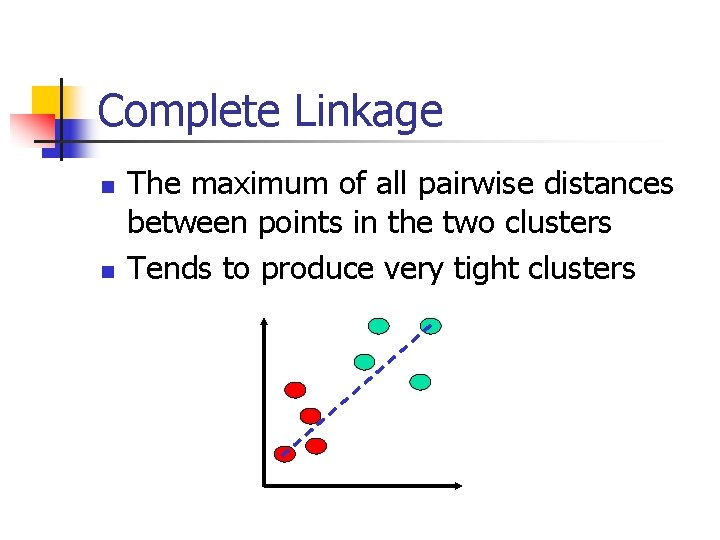Complete Linkage n n The maximum of all pairwise distances between points in the two clusters Tends to produce very tight clusters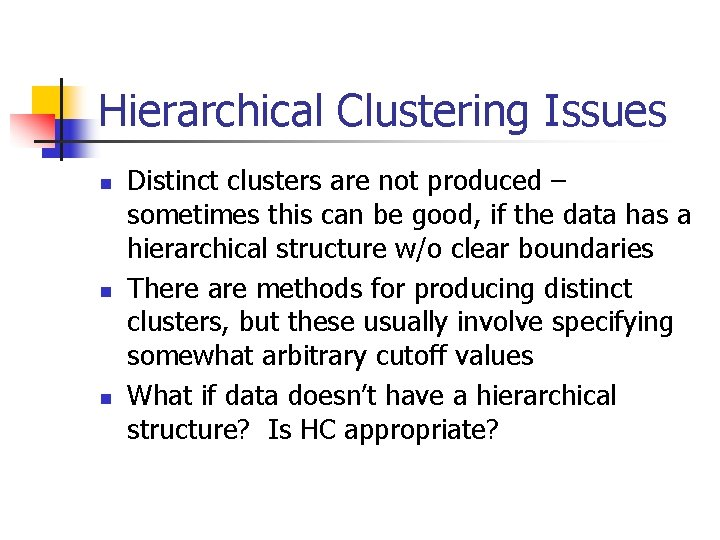Hierarchical Clustering Issues n n n Distinct clusters are not produced – sometimes this can be good, if the data has a hierarchical structure w/o clear boundaries There are methods for producing distinct clusters, but these usually involve specifying somewhat arbitrary cutoff values What if data doesn’t have a hierarchical structure? Is HC appropriate?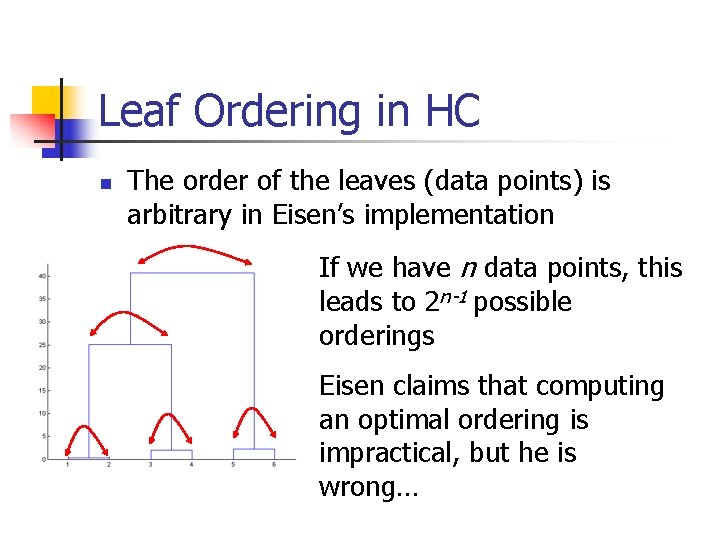Leaf Ordering in HC n The order of the leaves (data points) is arbitrary in Eisen’s implementation If we have n data points, this leads to 2 n-1 possible orderings Eisen claims that computing an optimal ordering is impractical, but he is wrong…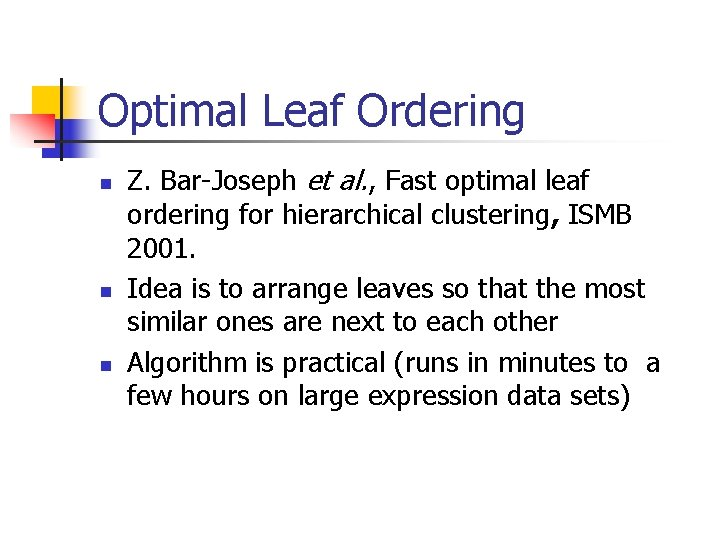Optimal Leaf Ordering n n n Z. Bar-Joseph et al. , Fast optimal leaf ordering for hierarchical clustering, ISMB 2001. Idea is to arrange leaves so that the most similar ones are next to each other Algorithm is practical (runs in minutes to a few hours on large expression data sets)Optimal Ordering Results Hierarchical clustering Input Optimal orderingK-means Clustering n n n Choose a number of clusters k Initialize cluster centers 1, … k n Could pick k data points and set cluster centers to these points n Or could randomly assign points to clusters and take means of clusters For each data point, compute the cluster center it is closest to (using some distance measure) and assign the data point to this cluster Re-compute cluster centers (mean of data points in cluster) Stop when there are no new re-assignments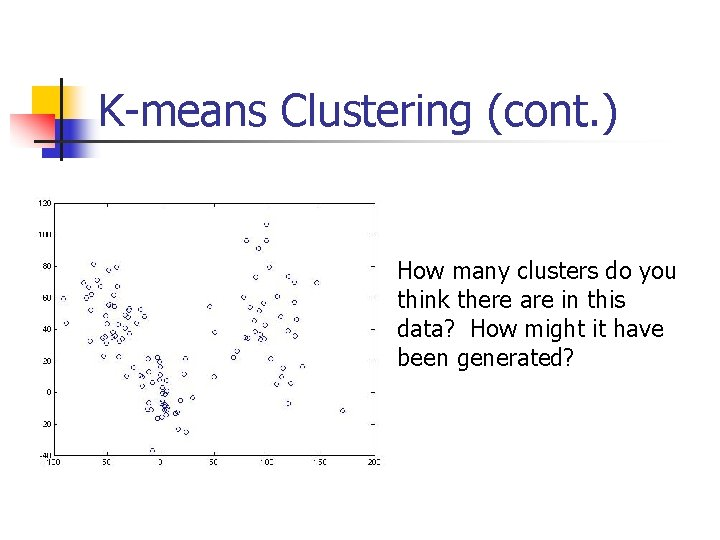K-means Clustering (cont. ) How many clusters do you think there are in this data? How might it have been generated?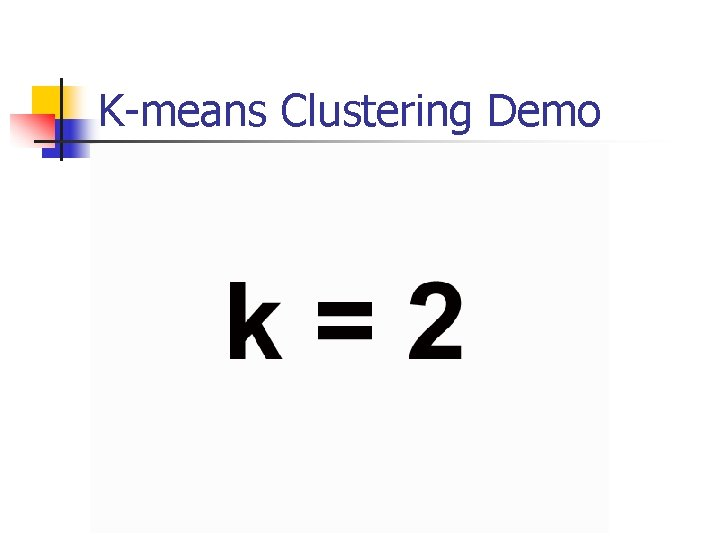K-means Clustering Demo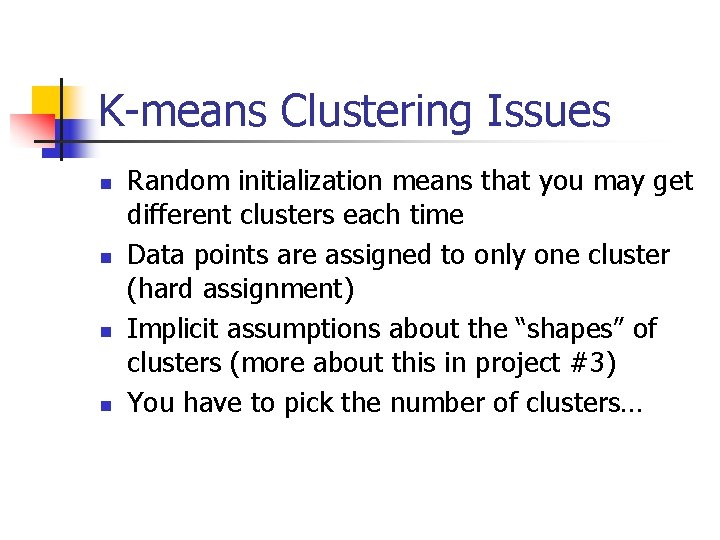K-means Clustering Issues n n Random initialization means that you may get different clusters each time Data points are assigned to only one cluster (hard assignment) Implicit assumptions about the “shapes” of clusters (more about this in project #3) You have to pick the number of clusters…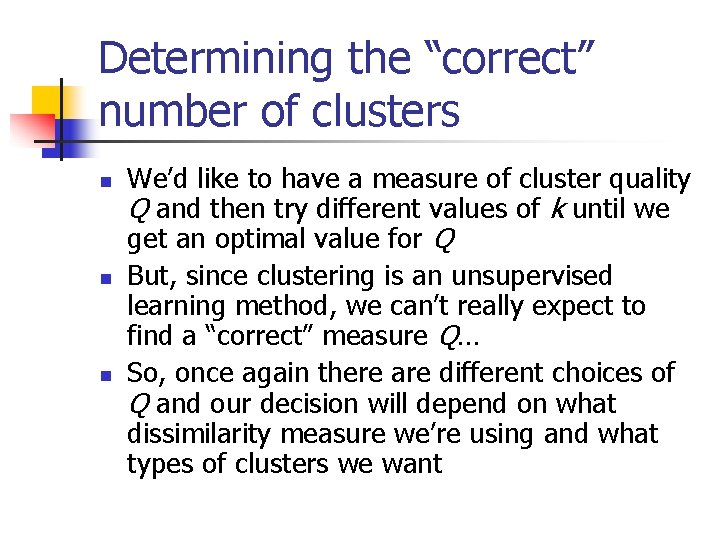Determining the “correct” number of clusters n n n We’d like to have a measure of cluster quality Q and then try different values of k until we get an optimal value for Q But, since clustering is an unsupervised learning method, we can’t really expect to find a “correct” measure Q… So, once again there are different choices of Q and our decision will depend on what dissimilarity measure we’re using and what types of clusters we want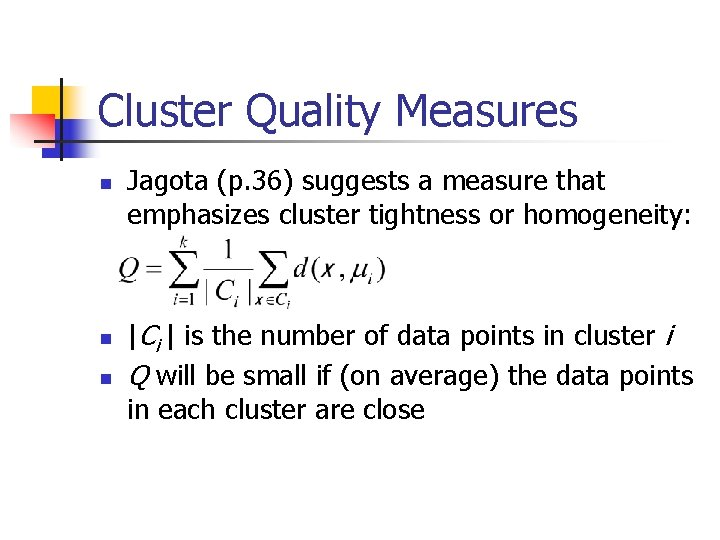Cluster Quality Measures n n n Jagota (p. 36) suggests a measure that emphasizes cluster tightness or homogeneity: |Ci | is the number of data points in cluster i Q will be small if (on average) the data points in each cluster are close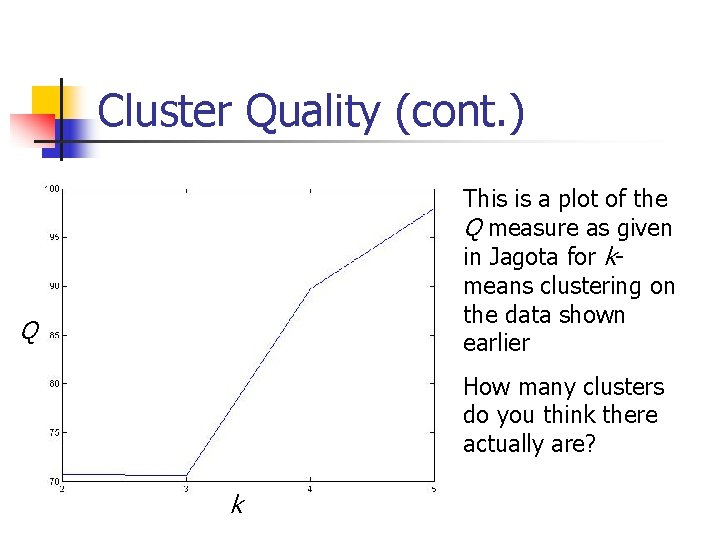Cluster Quality (cont. ) This is a plot of the Q measure as given in Jagota for kmeans clustering on the data shown earlier Q How many clusters do you think there actually are? k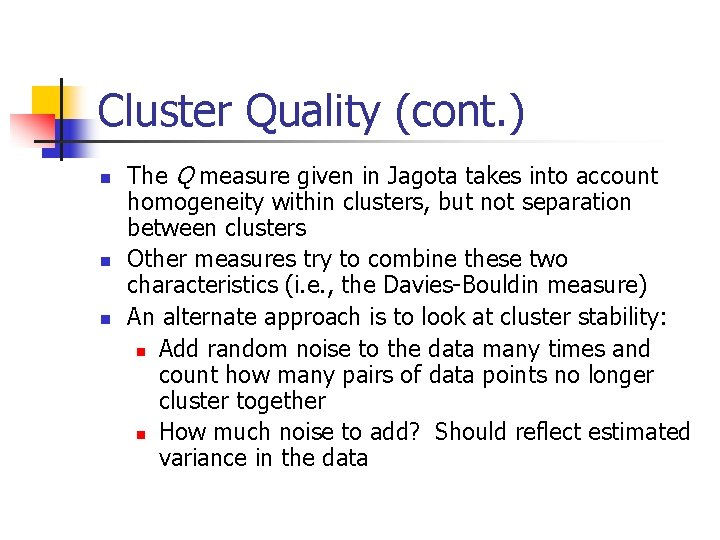Cluster Quality (cont. ) n n n The Q measure given in Jagota takes into account homogeneity within clusters, but not separation between clusters Other measures try to combine these two characteristics (i. e. , the Davies-Bouldin measure) An alternate approach is to look at cluster stability: n Add random noise to the data many times and count how many pairs of data points no longer cluster together n How much noise to add? Should reflect estimated variance in the dataSelf-Organizing Maps n n Based on work of Kohonen on learning/memory in the human brain As with k-means, we specify the number of clusters However, we also specify a topology – a 2 D grid that gives the geometric relationships between the clusters (i. e. , which clusters should be near or distant from each other) The algorithm learns a mapping from the high dimensional space of the data points onto the points of the 2 D grid (there is one grid point for each cluster)Self-Organizing Maps (cont. ) 10, 10 Grid points map to cluster means in high dimensional space (the space of the data points) 11, 11 Each grid point corresponds to a cluster (11 x 11 = 121 clusters in this example)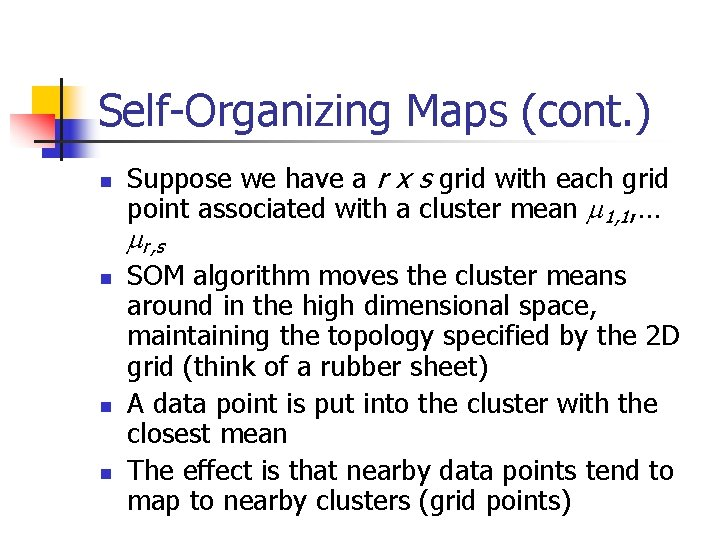Self-Organizing Maps (cont. ) n Suppose we have a r x s grid with each grid point associated with a cluster mean 1, 1, … r, s n n n SOM algorithm moves the cluster means around in the high dimensional space, maintaining the topology specified by the 2 D grid (think of a rubber sheet) A data point is put into the cluster with the closest mean The effect is that nearby data points tend to map to nearby clusters (grid points)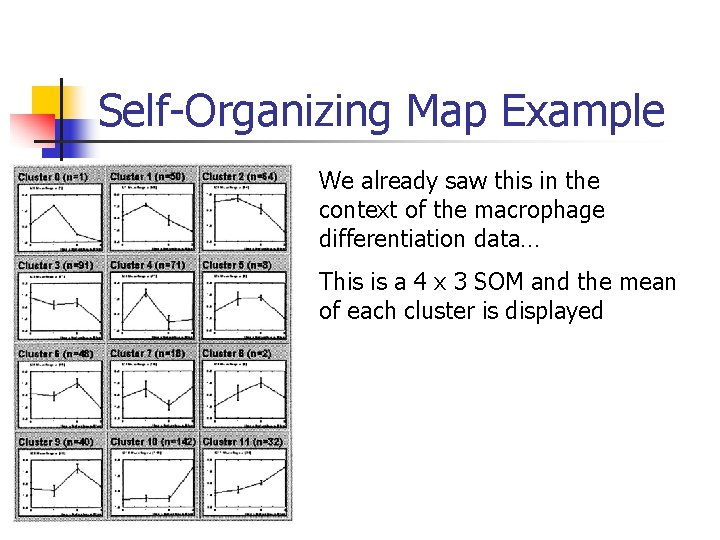Self-Organizing Map Example We already saw this in the context of the macrophage differentiation data… This is a 4 x 3 SOM and the mean of each cluster is displayed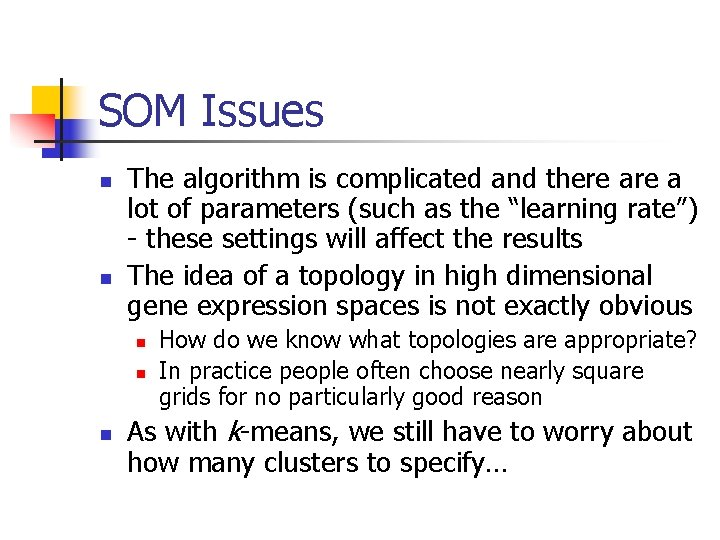SOM Issues n n The algorithm is complicated and there a lot of parameters (such as the “learning rate”) - these settings will affect the results The idea of a topology in high dimensional gene expression spaces is not exactly obvious n n n How do we know what topologies are appropriate? In practice people often choose nearly square grids for no particularly good reason As with k-means, we still have to worry about how many clusters to specify…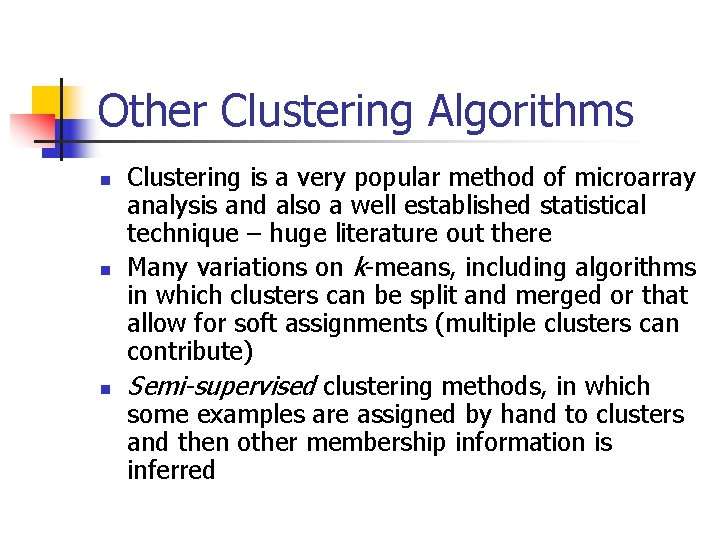Other Clustering Algorithms n n n Clustering is a very popular method of microarray analysis and also a well established statistical technique – huge literature out there Many variations on k-means, including algorithms in which clusters can be split and merged or that allow for soft assignments (multiple clusters can contribute) Semi-supervised clustering methods, in which some examples are assigned by hand to clusters and then other membership information is inferredParting thoughts: from Borges’ Other Inquisitions, discussing an encyclopedia entitled Celestial Emporium of Benevolent Knowledge “On these remote pages it is written that animals are divided into: a) those that belong to the Emperor; b) embalmed ones; c) those that are trained; d) suckling pigs; e) mermaids; f) fabulous ones; g) stray dogs; h) those that are included in this classification; i) those that tremble as if they were mad; j) innumerable ones; k) those drawn with a very fine camel brush; l) others; m) those that have just broken a flower vase; n) those that resemble flies at a distance. ”